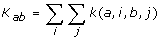Volume 3 Supplement 1

# Additive inductive learning in QSAR/QSPR studies and molecular modeling

In an ordinary inductive learning used in QSAR/QSPR studies a property y is approximated as a function of some fixed number of molecular descriptors:where x i is the value of the i-th descriptor, c j – is the values of the j-th learnable (adjustable) parameter, f – a non-linear function. Parameters c j are learned using some training set of compounds.

We define the additive inductive learning (AIL) as a process, in which a property y of a chemical object (such as chemical compound) consisting of s subobjects (such as atoms, bonds, fragments, etc) is approximated in an additive manner:where z ij is the value of the j-th descriptor for the i-th subobject. This definition can be extended to include several types of subobjects.

Due to the existence of many additive properties in chemistry and physics (the energy is the most prominent one), AIL is especially well suited for solving numerous problems in chemo- and bioinformatics, ranging from QSAR/QSPR studies up to molecular modeling, computing binding energies, etc.

We suggest two basic approaches to AIL: (i) the use of special additive neural networks (developed by us for this purpose), and (ii) the use of kernel-based approaches, such as the support vector machines, with a special type of kernels:where K ab is the kernel for the pair of objects a and b, while k(a, i, b, j) is the "microkernel" for the pair of the i-th subobject in a and the j-th subobject in b.

Several examples of using AIL in chemoinformatics and the prospects of its application in molecular modeling are considered.

Authors

## Rights and permissions

Reprints and Permissions

Baskin, I., Zhokhova, N., Palyulin, V. et al. Additive inductive learning in QSAR/QSPR studies and molecular modeling. Chemistry Central Journal 3 (Suppl 1), P25 (2009). https://doi.org/10.1186/1752-153X-3-S1-P25